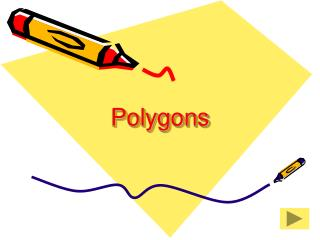DownloadDownload PresentationPolygons

# Polygons

Download Presentation## Polygons

- - - - - - - - - - - - - - - - - - - - - - - - - - - E N D - - - - - - - - - - - - - - - - - - - - - - - - - - -
##### Presentation Transcript

1. Polygons

2. Flow Chart End show

3. Polygon A polygon is a two-dimensional geometric figure with the following characteristics: • It is made of straight line segments • Each segment touches exactly two other segments, on at teach of its endpoints • It is closed – it divides the plane into tow distinct region, one inside and the other outside the polygon Back

4. Rectangle Square Rhombus Diamond Trapezoid Parallelogram Quadrilateral A quadrilateral is a polygon with exactly 4 sides. Back

5. Rectangle Square Rhombus Parallelogram Diamond Parallelogram A parallelogram is a quadrilateral that has two pairs of opposite sides that are parallel. Back

6. Rectangle A rectangle is a quadrilateral with four right angles. All rectangles are: Quadrilaterals and Parallelograms Back

7. Square A square is a quadrilateral with sides that are congruent and angles that are all the same size. All squares are: Quadrilaterals, Parallelograms and Rectangles Back

8. Rhombus A rhombus is a quadrilateral with sides that are congruent. All rhombuses are: Quadrilaterals and Parallelograms Back

9. rhombus square Diamond A diamond is a square or rhombus turned on a point. All diamonds are: Quadrilaterals and Parallelograms Back

10. Trapezoid A trapezoid is a quadrilateral that has one pair of opposite sides that are parallel. All trapezoids are: Quadrilaterals Back

11. Triangle A triangle is a polygon with exactly 3 sides. Back

12. Pentagon A pentagon is a polygon with exactly 5 sides. Back

13. Hexagon A hexagon is a polygon with exactly 6 sides. Back

14. Heptagon A Heptagon is a polygon with exactly 7 sides. Back

15. Octagon An Octagon is a polygon with exactly 8 sides. Back

16. The End